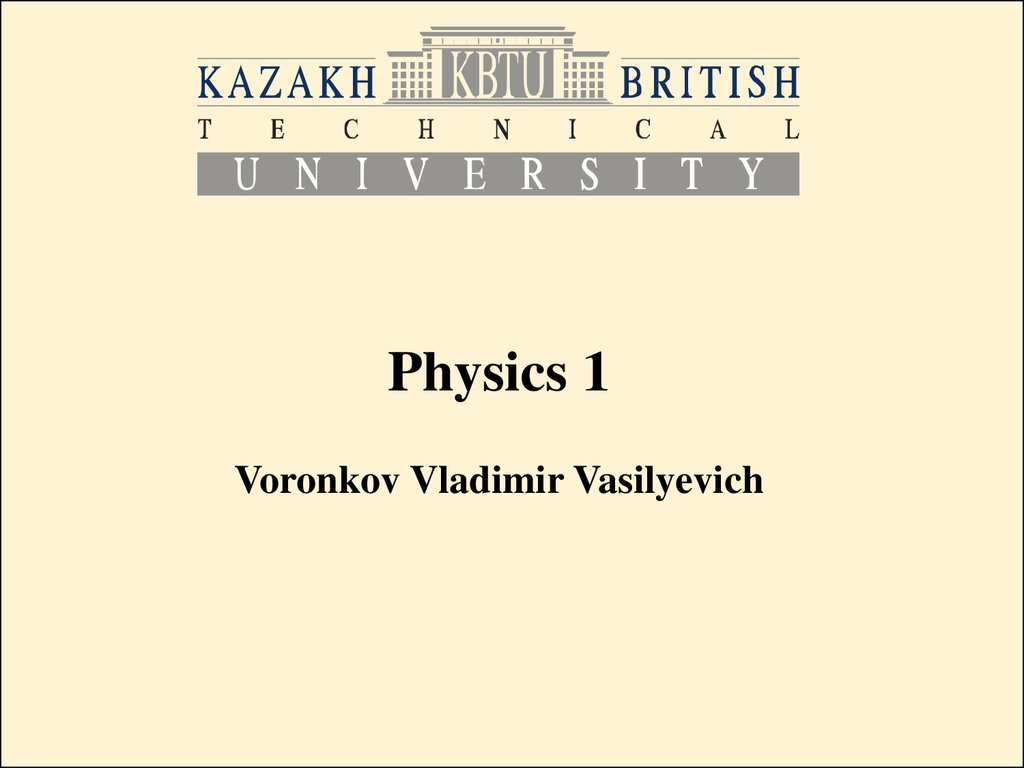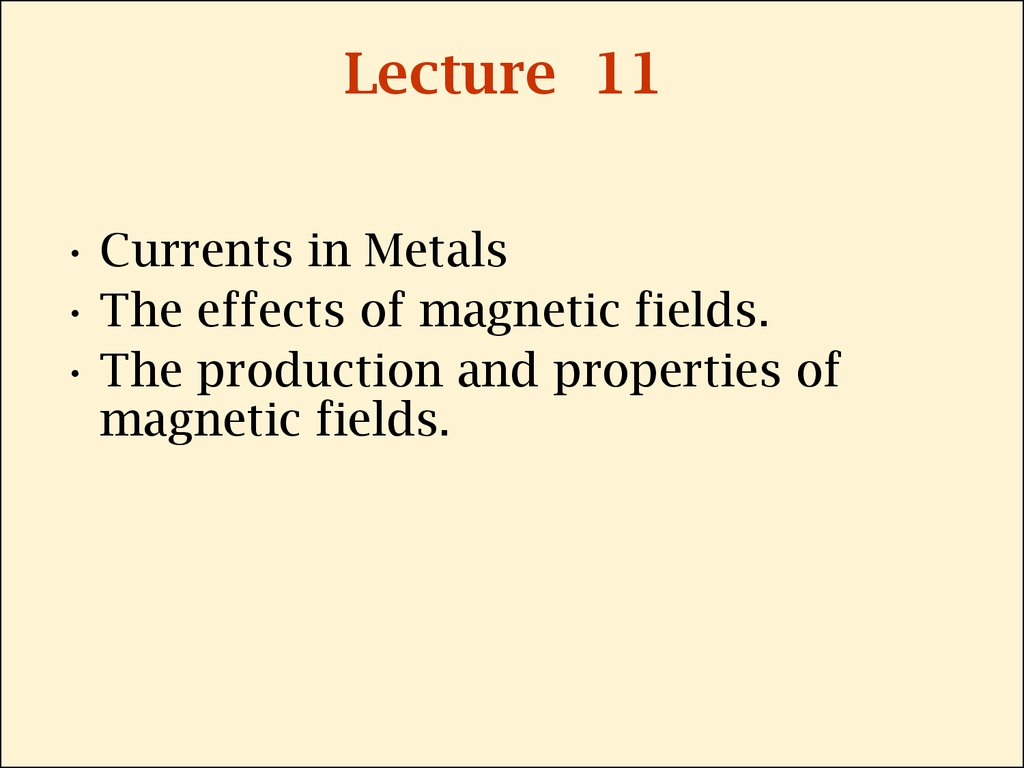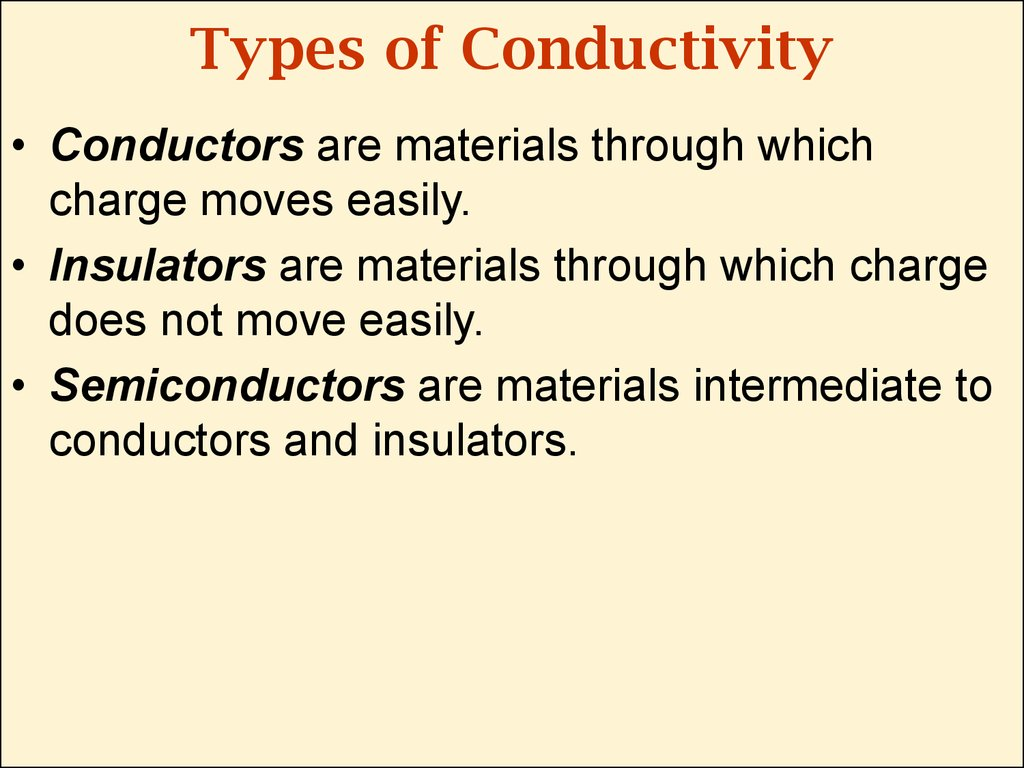# Currents in мetals

Physics 1

## 2. Lecture 11

• Currents in Metals
• The effects of magnetic fields.
• The production and properties of
magnetic fields.

## 3. Types of Conductivity

• Conductors are materials through which
charge moves easily.
• Insulators are materials through which charge
does not move easily.
• Semiconductors are materials intermediate to
conductors and insulators.

## 4. Drift speed of electrons

• There is a zigzag motion of an electron in
a conductor. The changes in direction are
the result of collisions between the
electron and atoms in the conductor. The
net motion – drift speed of the electron is
opposite the direction of the electric field.

## 5.

• So when we consider electric current as a
flow of electrons:
in reality there happens zigzag motion of
free electrons in the metal:

## 6. Current in metals

• Every atom in the metallic crystal gives up one
or more of its outer electrons. These electrons
are then free to move through the crystal,
colliding at intervals with stationary positive
ions, then the resistivity is:
r = m/(ne2t)
n - the number density of free electrons,
m and e – mass and charge of electron,
t – average time between collisions.

## 7. Resistivity

• A conductor with current:
• Current density:
• I – electric current
• A – the cross-sectional area of the
conductor
• vd – drift speed
E = rJ
r - resistivity

## 8. Conductivity

• A current density J and an electric field E
are established in a conductor whenever a
potential difference is maintained across the
conductor:
s is conductivity:
s = 1/ r.

## 9. Ohm’s law again

• For many materials (including most metals),
the ratio of the current density to the electric
field is a constant s that is independent of the
electric field producing the current:
J = sE

## 10. Magnets

• A single magnetic pole has never been isolated.
Magnetic poles are always found in pairs.
• The direction of magnetic field is from the North
pole to the South pole of a magnet.

## 11. Magnet Poles

• Magnet field lines connect
unlike poles.
• Magnet field lines repels from
like poles.

## 12. Magnet Force

• The magnitude FB of the magnetic force exerted on the particle
is proportional to the charge q and to the speed v of the
particle.
• The magnitude and direction of FB depend on the velocity of the
particle and on the magnitude and direction of the magnetic
field B.
• When a charged particle moves parallel to the magnetic field
vector, the magnetic force acting on the particle is zero.
• When the particle’s velocity vector makes any angle Q 0 with
the magnetic field, the magnetic force acts in a direction
perpendicular to both v and B.
• The magnetic force exerted on a positive charge is in the
direction opposite the direction of the magnetic force exerted
on a negative charge moving in the same direction.
• The magnitude of the magnetic force exerted on the moving
particle is proportional to sin Q, where Q is the angle the
particle’s velocity vector makes with the direction of B.

## 13.

The text in the previous slide can be summarized as:
So the units for B are:
The magnetic force is
perpendicular to both v and B.
FB=qVBsinQ

## 14. Direction of FB

• Right hand rule:
The fingers point in the direction
of v, with B coming out of your
palm, so that you can curl your
fingers in the direction of B. The
direction of
, and the force
on a positive charge, is the
direction in which the thumb
points.

## 15. Magnetic field direction

• Magnetic field lines coming out
of the paper are indicated by
dots, representing the tips of
arrows coming outward.
• Magnetic field lines going into
the paper are indicated by
crosses, representing the
feathers of arrows going
inward.

## 16. Magnetic Force on a Current

• Magnetic force is exerted on a single charge moving in
a magnetic field. A current-carrying wire also
experiences a force when placed in a magnetic field.
This follows from the fact that the current is a
collection of many charged particles in motion; hence,
the resultant force exerted by the field on the wire is
the vector sum of the individual forces exerted on all
the charges making up the current. The force
exerted on the particles is transmitted to the wire
when the particles collide with the atoms making up
the wire.

## 17.

• n is the number density
of charged particles q
• vd is the drift speed of q
• A – area of the segment
• L – the length of the
segment
• Then AL is the volume
of the segment, and
• nAL is the number of charged particles q.
• Then the net force acting on all moving
charges is:

## 18. Arbitrary shaped wire

• The force on a small segment of an
arbitrary shaped wire is:
• The total force is:
• a and b are the end points of the wire.

## 19. Curved Wire in a Uniform Magnetic field

as B is uniform:
• The magnetic force on a curved currentcarrying wire in a uniform magnetic field is
equal to that on a straight wire connecting
the end points and carrying the same current.

## 20. Magnetic force on a straight wire

So, the force on a straight wire in a uniform
magnetic field is:
is a vector multiplication.
• Where L is a vector that points in the direction
of the current I and has a magnitude equal to
the length L of the segment. This expression
applies only to a straight segment of wire in a
uniform magnetic eld.

## 21. Loop Wire in a Uniform Magnetic field

• The net magnetic force
acting on any closed
current loop in a uniform
magnetic field is zero:
• Then the net force is zero:
FB=0

## 22. Current Loop Torque in a Uniform Magnetic Field

rectangular loop in a
uniform magnetic field.
Sides 1 and 3 are parallel
to magnetic field, so only
sides 2 and for experiences
magnetic forces.
- Magnet forces, acting on
sides 2 and 4 create a
torque on the loop.

## 23.

When the magnetic field is
parallel to the plane of the
loop, the maximal torque on
the loop is:
ab is the area of the loop A:

## 24.

the
A and B is
then:
When the loop is not
parallel to the
magnetic field, i.e.
angle between
Q < 90°
So the torque on a loop in a uniform magnetic field
is:
This formula is correct not only for a rectangular
loop, but for a planar loop of any shape.

## 25. Area Vector

In formula for torque
we have vector A:
- Its direction is perpendicular
to the plane of the loop,
- its magnitude is equal to the
area of the loop.
• We determine the direction of A using the right-hand
rule. When you curl the fingers of your right hand in
the direction of the current in the loop, your thumb
points in the direction of A.

## 26. Right – hand rule for loop

The direction of the
magnetic moment is the same as
the direction of A.

## 27. Magnetic Moment

• The vector product IA is defined to be the
magnetic dipole moment m (often simply called
the “magnetic moment”) of the current loop:
• Then the torque on a current-carrying loop is:

## 28. Potential Energy of a Magnetic Moment

• The potential energy of a system having
magnetic dipole m in the magnetic field B
is:
• Here we have scalar product m B. Then the
lowest energy is when m points as B, the
highest energy is when m points opposite B:

## 29. Motion of a Charged Particle in a Uniform Magnetic Field

When the velocity of a
charged particle is
perpendicular to a uniform
magnetic field, the particle moves in a circular
path in a plane perpendicular to B. The
magnetic force FB acting on the charge is
always directed toward the center of the circle.

## 30.

Using the obtained formula
we get the angular velocity
here v is perpendicular to B.

## 31. Lorentz Force

• In the presence of E and B, the force
acting on a charged particle is:
here q is the charge of the particle,
v – the speed of the particle,
E – electric field vector
B – magnetic field vector

## 32. The Hall Effect

• When a current-carrying conductor is
placed in a magnetic field, a potential
difference is generated in a direction
perpendicular to both the current and the
magnetic field.

## 33.

the magnetic force
exerted on the carriers
has magnitude qvdB.
this force is balanced
by the electric force qEH:
d is the width of the conductor:
n – charge density:
then we obtain the Hall voltage:
.vd - charge carrier drift speed.

## 34.

Using that A=td – cross sectional area of the conductor,
t – thickness of the conductor we can obtain:
RH is the Hall coefficient:
RH = 1/(nq)

## 35.

When the charge carriers in
a Hall-effect apparatus are
negative, the upper edge of
the conductor becomes
negatively charged, and c is
at a lower electric potential
than a.
When the charge carriers are
positive, the upper edge
becomes positively charged,
and c is at a higher potential
than a.

• Magnetic field
B
• Electric Field
E
• Number density
n
• Torque
t
T= N*s/(C*m)
T= N/(A*m)
V/m=N/C
1/m3
N*m